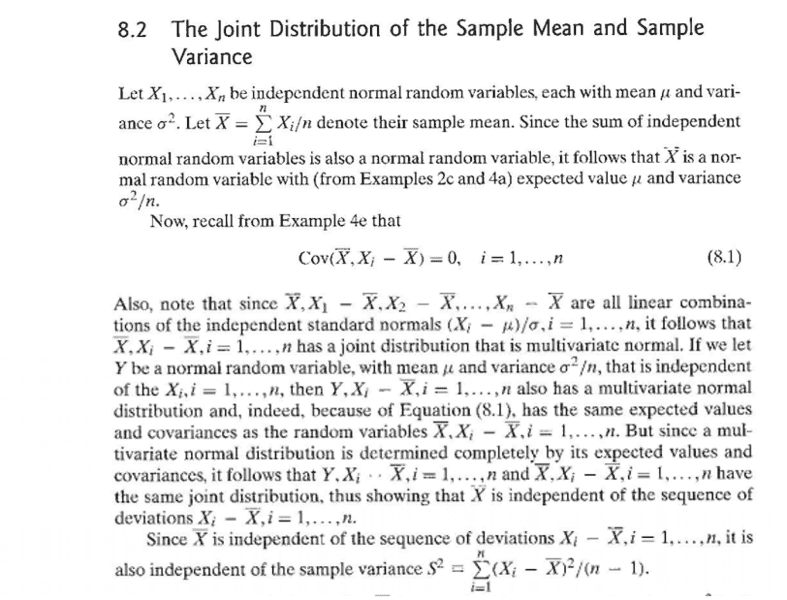# Proving a multivariate normal distribution by the moment generating function

• I
• Torgny

#### TorgnyI have proved (8.1). However I am trying to prove that

##\bar{X},X_i-\bar{X},i=1,...,n## has a joint distribution that is multivariate normal. I am trying to prove it by looking at the moment generating function:

##E(e^{t(X_i-\bar{X})}=E(e^{tX_i})E(e^{-\frac{t}{n}\sum_{i=1}^n X_i})##

I am trying to use the moment generating function because there is only one moment generating function for a given probability distribution and this also holds for multivariate distributions. But I fail at obtaining a moment generating function. The mgf to ##E(e^{tX_i})## is simply the mgf to the normal distribution but I can't get a moment generating function to ##E(e^{-\frac{t}{n}\sum_{i=1}^n X_i})## which from the answer in the text i guess should be a multivariate normal distribution.

Can someone help out?

#### Attachments

Thanks! But what do I do with:

##-\frac{1}{n}##
in

##E(e^{tX_i})E(e^{-\frac{t}{n}\sum_{i=1}^n X_i})##

I can see that the rest follows the relation:

##\varphi_{X+Y}=\varphi_{X}\varphi_{Y}##

Last edited by a moderator:
I'm not quite sure of your motivation for what you're trying to do here. Moment generating functions can be useful, but frequently are not needed -- this is one of those cases.

- - - -
It seems to me there are only two building blocks.

1.) "since the sum of independent normal random r.v.'s is a normal r.v." This holds -- the sum of finitely many independent normal rvs is normal rv. It is enough to prove that the convolution of two independent normals is a normal, and induct from there. Moment generating functions aren't needed here.

2.) the fact that the joint distribution of normal r.v.'s is a multivariate normal r.v.

If you have proven 1, apply it such that ##Y_i := X_i - \overline{X}## must be a normal r.v. Why? Because it is the convolution of ##X_i## with 1 piece (with ##\frac{1}{n}## weighting) that is strictly dependent on ##X_i## -- and in fact is a negative scaled down, version of ##X_i##. This has the effect of rescaling ##X_i##, but its still a normal r.v.. Then the resulting##X_i## is convolved with ##(n-1)## other independent normals (though each value of said n-1 normals is rescaled by -1). So repeatedly apply part 1 here, and the result is a normal r.v.

Then apply 2.

I'm not quite sure of your motivation for what you're trying to do here. Moment generating functions can be useful, but frequently are not needed -- this is one of those cases.

- - - -
It seems to me there are only two building blocks.

1.) "since the sum of independent normal random r.v.'s is a normal r.v." This holds -- the sum of finitely many independent normal rvs is normal rv. It is enough to prove that the convolution of two independent normals is a normal, and induct from there. Moment generating functions aren't needed here.

2.) the fact that the joint distribution of normal r.v.'s is a multivariate normal r.v.

If you have proven 1, apply it such that ##Y_i := X_i - \overline{X}## must be a normal r.v. Why? Because it is the convolution of ##X_i## with 1 piece (with ##\frac{1}{n}## weighting) that is strictly dependent on ##X_i## -- and in fact is a negative scaled down, version of ##X_i##. This has the effect of rescaling ##X_i##, but its still a normal r.v.. Then the resulting##X_i## is convolved with ##(n-1)## other independent normals (though each value of said n-1 normals is rescaled by -1). So repeatedly apply part 1 here, and the result is a normal r.v.

Then apply 2.

Thanks for the insight. However I believe that I will not get an accepted answer unless I prove it mathematically. For 1) I can prove it as noted above like this:

##E(e^{X+Y})=E(e^X)E(e^Y)##

But I don't know how to prove the things you adress afterwards with equations.

Thanks for the insight. However I believe that I will not get an accepted answer unless I prove it mathematically. For 1) I can prove it as noted above like this:

##E(e^{X+Y})=E(e^X)E(e^Y)##

But I don't know how to prove the things you adress afterwards with equations.

They also show the convolution of two normal r.v.'s directly here:

https://ocw.mit.edu/courses/electri...s-convolution-correlation/MIT6_041F10_L11.pdf

(MIT is not very big on moment generating functions. )
- - - -

To be clear the outline I gave was mathematical. You'd just need to recut it into a couple of lemmas, then carefully use induction in the main argument. The underlying idea that comes up over and over (in both part 1 and part 2) is that convolving a random variable with a scaled down version of itself is just a rescaling. And convolving a normal r.v. with an independent normal r.v. results in a normal r.v.

There would only be one or two equations here -- and it has a linear algebra flair in that everything we're interested in is written as a linear combination of a scaled version of identical random normals (i.e. rescaling) and independent normals. It's actually a very simple idea.

- - - -
You seem to be quite keen on using MGFs which is not how I'd look at this. Good luck.